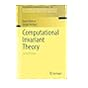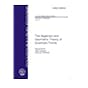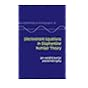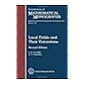Normal view MARC view ISBD view

# A primer on mapping class groups / Benson Farb, Dan Margalit.

Material type:TextSeries: Princeton mathematical series.Publisher: Princeton, N.J. ; Woodstock : Princeton University Press, c2012Description: xiv, 472 p., USD 75.00 ill. ; 24 cm.ISBN: 9780691147949 (hbk.) :; 0691147949 (hbk.) :.Subject(s): Mappings (Mathematics) | Class groups (Mathematics)DDC classification: 512.74 Summary: "The study of the mapping class group Mod(S) is a classical topic that is experiencing a renaissance. It lies at the juncture of geometry, topology, and group theory. This book explains as many important theorems, examples, and techniques as possible, quickly and directly, while at the same time giving full details and keeping the text nearly self-contained. The book is suitable for graduate students.The book begins by explaining the main group-theoretical properties of Mod(S), from finite generation by Dehn twists and low-dimensional homology to the Dehn-Nielsen-Baer theorem. Along the way, central objects and tools are introduced, such as the Birman exact sequence, the complex of curves, the braid group, the symplectic representation, and the Torelli group. The book then introduces Teichm©ơller space and its geometry, and uses the action of Mod(S) on it to prove the Nielsen-Thurston classification of surface homeomorphisms. Topics include the topology of the moduli space of Riemann surfaces, the connection with surface bundles, pseudo-Anosov theory, and Thurston's approach to the classification"--
Item type Current location Call number Status Date due Barcode Item holds
Book Chennai Mathematical Institute
General Stacks
512.74 FAR (Browse shelf) Available 8537
Total holds: 0
##### Browsing Chennai Mathematical Institute Shelves , Shelving location: General Stacks Close shelf browser512.74 DER Computational invariant theory / 512.74 ELM The algebraic and geometric theory of quadratic forms / 512.74 EVE Discriminant equations in Diophantine number theory / 512.74 FAR A primer on mapping class groups / 512.74 FES Local fields and their extensions / 512.74 GER Basic quadratic forms / 512.74 GOL Automorphic forms and L-functions for the group GL(n, R) /

Formerly CIP. Uk

Includes bibliographical references and index.

"The study of the mapping class group Mod(S) is a classical topic that is experiencing a renaissance. It lies at the juncture of geometry, topology, and group theory. This book explains as many important theorems, examples, and techniques as possible, quickly and directly, while at the same time giving full details and keeping the text nearly self-contained. The book is suitable for graduate students.The book begins by explaining the main group-theoretical properties of Mod(S), from finite generation by Dehn twists and low-dimensional homology to the Dehn-Nielsen-Baer theorem. Along the way, central objects and tools are introduced, such as the Birman exact sequence, the complex of curves, the braid group, the symplectic representation, and the Torelli group. The book then introduces Teichm©ơller space and its geometry, and uses the action of Mod(S) on it to prove the Nielsen-Thurston classification of surface homeomorphisms. Topics include the topology of the moduli space of Riemann surfaces, the connection with surface bundles, pseudo-Anosov theory, and Thurston's approach to the classification"--